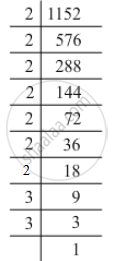Advertisement Remove all ads

# P Find the Smallest Number by Which 1152 Must Be Divided So that It Becomes a Perfect Square. Also, Find the Number Whose Square is the Resulting Number. - Mathematics

Find the smallest number by which 1152 must be divided so that it becomes a perfect square. Also, find the number whose square is the resulting number.

Advertisement Remove all ads

#### Solution

Prime factorisation of 1152:
1152 = 2 x 2 x 2 x 2 x 2 x 2 x 2 x 3 x 3Grouping them into pairs of equal factors:
1152 = (2 x 2) x (2 x 2) x (2 x 2) x (3 x 3) x 2
The factor, 2 at the end is not paired. For a number to be a perfect square, each prime factor has to be paired. Hence, 1152 must be divided by 2 for it to be a perfect square.
The resulting number would be (2 x 2) x (2 x 2) x (2 x 2) x (3 x 3).
Furthermore, we have:
(2 x 2) x (2 x 2) x (2 x 2) x (2 x 2) x (3 x 3) = (2 x 2 x 2 x 3) x (2 x 2 x 2 x 3)
Hence, the number whose square is the resulting number is:
2 x 2 x 2 x 3 = 24

Is there an error in this question or solution?
Advertisement Remove all ads

#### APPEARS IN

RD Sharma Class 8 Maths
Chapter 3 Squares and Square Roots
Exercise 3.1 | Q 13 | Page 5
Advertisement Remove all ads

#### Video TutorialsVIEW ALL 

Advertisement Remove all ads
Share
Notifications

View all notifications

Forgot password?
Course